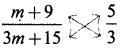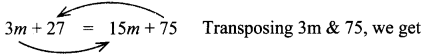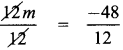Tamilnadu State Board New Syllabus Samacheer Kalvi 8th Maths Guide Pdf Chapter 3 Algebra Ex 3.6 Text Book Back Questions and Answers, Notes.

## Tamilnadu Samacheer Kalvi 8th Maths Solutions Chapter 3 Algebra Ex 3.6

Question 1.
Fill in the blanks:
(i) The value of x in the equation x + 5 = 12 is ________ .
7
Hint:
Given, x + 5 = 12
x = 12 – 5 = 7 (by transposition method)
Value of x is 7(ii) The value of y in the equation y – 9 = (-5) + 7 is ________ .
11
Hint:
Given, y – 9 = (-5) + 7
y – 9 = 7 – 5 (re-arranging)
y – 9 = 2
∴ y = 2 + 9 = 11 (by transposition method)

(iii) The value of m in the equation 8m = 56 is ________ .
7
Hint:
Given, 8m = 56
Divided by 8 on both sides
$$\frac{8 \times m}{8}=\frac{56}{8}$$
∴ m = 7(iv) The value of p in the equation $$\frac{2 p}{3}$$ = 10 is ________ .
15
Hint:
Given, $$\frac{2 p}{3}$$ = 10
Multiplying by 3 on both sides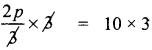Dividing by 2 on both sides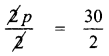∴ p = 15

(v) The linear equation in one variable has ________ solution.
one

Question 2.
Say True or False.
(i) The shifting of a number from one side of an equation to other is called transposition.
True

(ii) Linear equation in one variable has only one variable with power 2.
False
[Linear equation in one variable has only one variable with power one – correct statement]Question 3.
Match the following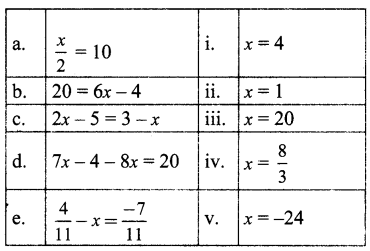(A) (i),(ii), (iv) ,(iii),(v)
(B) (iii), (iv), (i) ,(ii), (v)
(C) (iii),(i) ,(iv), (v), (ii)
(D) (iii) , (i) , (v) ,(iv) ,(ii)
(C) (iii),(i) ,(iv), (v), (ii)

a. $$\frac{x}{2}$$ = 10, multiplying by 2 on both sides, we get
$$\frac{x}{2}$$ × 2 = 10 × 2 ⇒ x = 20

b. 20 = 6x – 4 by transposition ⇒ 20 + 4 =6x
6x = 24 dividing by 6 on both sides,
$$\frac{6 x}{6}=\frac{24}{6}$$ ⇒ x = 4

c. 2x – 5 = 3 – x
By transposing the variable ‘x’, we get
2x – 5 + x = 3
by transposing – 5 to other side,
2x + x = 3 + 5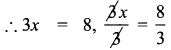∴ x = $$\frac{8}{3}$$

d. 7x – 4 – 8x = 20
by transposing – 4 to other side,
7x – 8x = 20 + 4
– x = 24
∴ x = – 24

e. $$\frac{4}{11}-x=\frac{-7}{11}$$
Transposing $$\frac{4}{11}$$ to other side,
– x = $$\frac{-7}{11} \frac{-4}{11}=\frac{-7-4}{11}=\frac{-11}{11}$$ = – 1
∴ – x = – 1 ⇒ x = 1Question 4.
Find x:
(i) $$\frac{2 x}{3}-4=\frac{10}{3}$$
Transposing – 4 to other side, it becomes + 4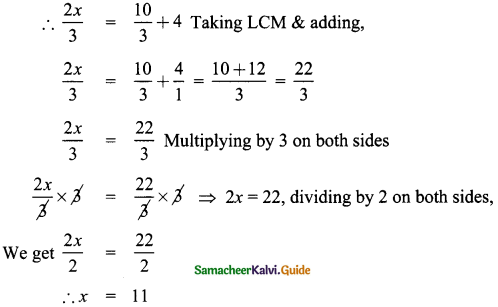(ii) $$y+\frac{1}{6}-3 y=\frac{2}{3}$$
Transposing  to the other side,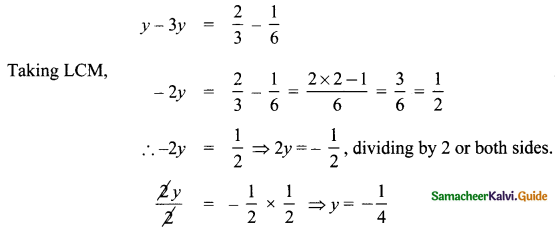(iii) $$\frac{1}{3}-\frac{x}{3}=\frac{7 x}{12}+\frac{5}{4}$$
Transposing $$\frac{-x}{3}$$ to the other side, it becomes + $$\frac{x}{3}$$
∴ $$\frac{1}{3}=\frac{7 x}{12}+\frac{5}{4}+\frac{x}{3}$$
Transposing $$\frac{5}{4}$$ to the other side, it becomes $$\frac{-5}{4}$$
$$\frac{1}{3}-\frac{5}{4}=\frac{7 x}{12}+\frac{x}{3}$$
Multiply by 12 throughout [we look at the denominators 3, 4, 12, 3 and take the LCM, which is 12]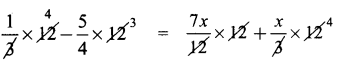4 – 15 = 7x + x × 4
– 11 = 7x + 4x
11x = – 11
x = -1

Question 5.
Find x
(i) -3(4x + 9) = 21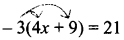Expanding the bracket,
-3 × 4 + (-3) × 9 = 21
∴ -12x + (-27) = 21
– 12x – 27 = 21
Transposing – 27 to other side, it becomes +27
– 12 x = 21 + 27 = 48
∴ – 12x = 48 ⇒ 12x = – 48
Dividing by 12 on both sides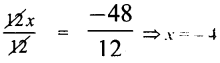(ii) 20 – 2 (5 – p) = 8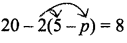Expanding the bracket,
20 – 2 × 5 – 2 × (-p) = 8
20 – 10 + 2p = 8
(- 2 × – p = 2p)
10 + 2p = 8 transposing lo to other side
2p = 8 – 10 = – 2
∴ 2p = – 2
∴ p = – 1

(iii) (7x – 5) – 4(2 + 5x) = 10(2 – x)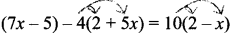Expanding the brackets,
7x – 5 – 4 × 2 – 4 × 5x = 10 × 2 + 10 × (-x)
7x – 5 – 8 – 20x = 20 – 10x
7x – 13 – 20x = 20 – 10x
Transposing 10x & – 13, we get
7x – 13 – 20x + 10x = 20
7x – 20x + 10x = 20 + 13, Simplifying,
– 3x = 33
3x = – 33
x = $$\frac{-33}{3}$$ = – 11
x = – 11Question 6.
Find x and m:
(i) $$\frac{3 x-2}{4}-\frac{(x-3)}{5}=-1$$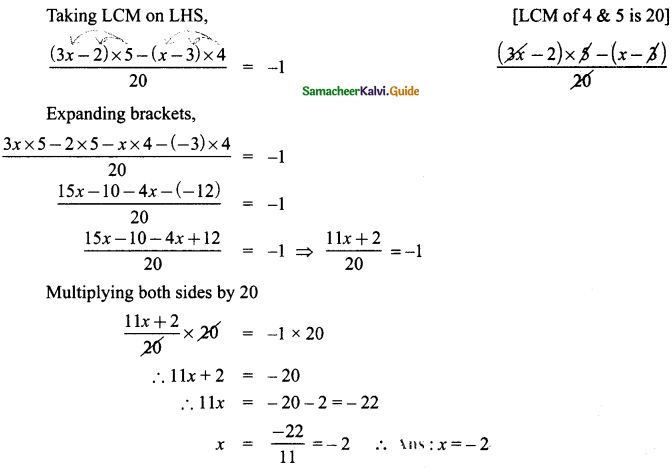(ii) $$\frac{m+9}{3 m+15}=\frac{5}{3}$$